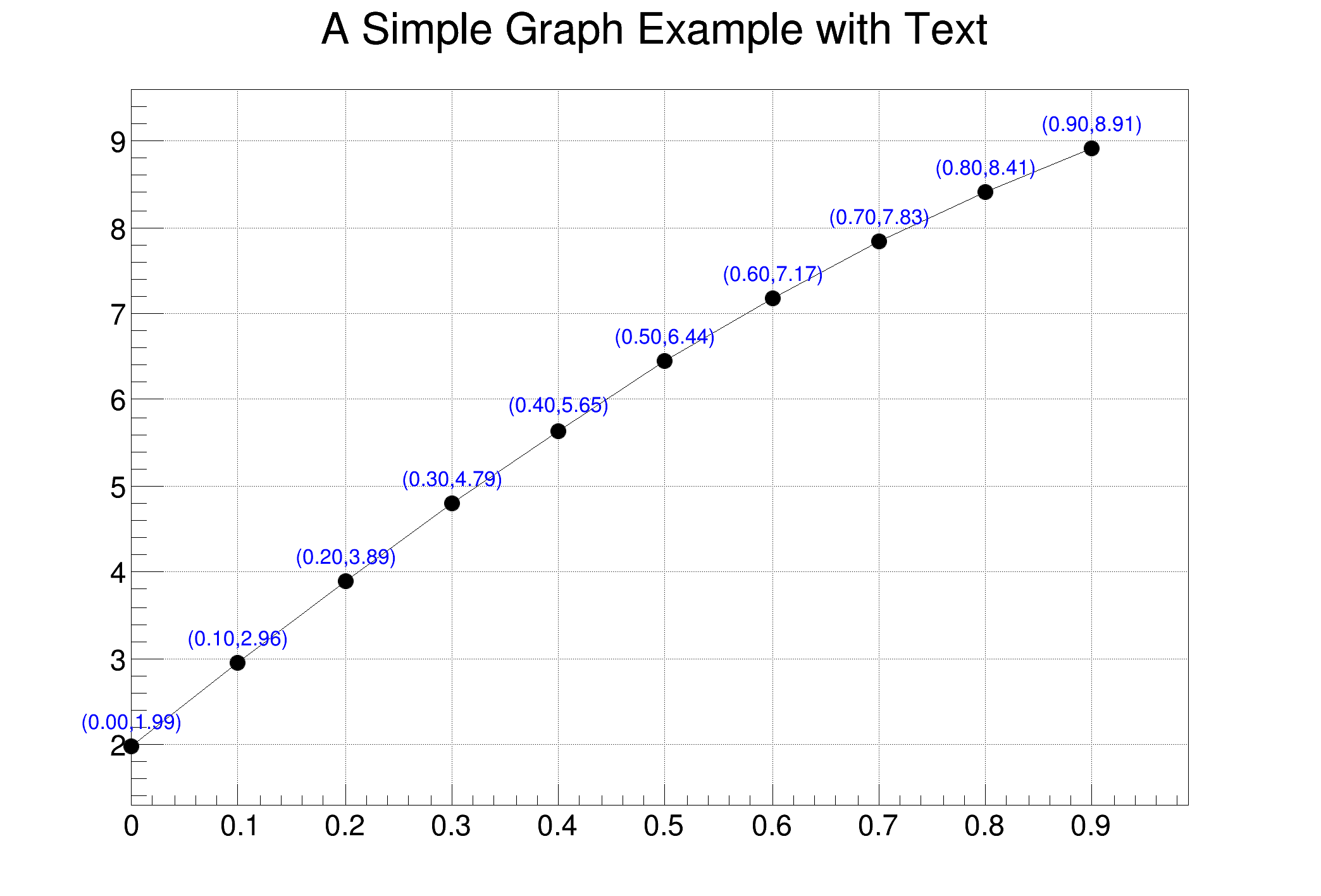ROOT   Reference Guidegraphtext.C File Reference

## Detailed DescriptionDraw a graph with text attached to each point.

The text is drawn in a TExec function attached to the TGraph, therefore if the a graph's point is moved interactively, the text will be automatically updated.void graphtext() {
TCanvas *c = new TCanvas("c","A Simple Graph Example with Text",700,500);
c->SetGrid();
const Int_t n = 10;
auto gr = new TGraph(n);
gr->SetTitle("A Simple Graph Example with Text");
auto ex = new TExec("ex","drawtext();");
for (Int_t i=0;i<n;i++) {
x = i*0.1;
y = 10*sin(x+0.2);
gr->SetPoint(i,x,y);
}
gr->Draw("ALP");
}
void drawtext()
{
Int_t i,n;
l.SetTextSize(0.025);
l.SetTextFont(42);
l.SetTextAlign(21);
l.SetTextColor(kBlue);
n = g->GetN();
for (i=0; i<n; i++) {
g->GetPoint(i,x,y);
l.PaintText(x,y+0.2,Form("(%4.2f,%4.2f)",x,y));
}
}
#define c(i)
Definition: RSha256.hxx:101
#define g(i)
Definition: RSha256.hxx:105
int Int_t
Definition: RtypesCore.h:45
double Double_t
Definition: RtypesCore.h:59
@ kBlue
Definition: Rtypes.h:66
double sin(double)
char * Form(const char *fmt,...)
virtual void SetMarkerStyle(Style_t mstyle=1)
Set the marker style.
Definition: TAttMarker.h:40
The Canvas class.
Definition: TCanvas.h:23
TExec is a utility class that can be used to execute a C++ command when some event happens in a pad.
Definition: TExec.h:28
A TGraph is an object made of two arrays X and Y with npoints each.
Definition: TGraph.h:41
virtual void SetPoint(Int_t i, Double_t x, Double_t y)
Set x and y values for point number i.
Definition: TGraph.cxx:2284
virtual void SetTitle(const char *title="")
Change (i.e.
Definition: TGraph.cxx:2339
virtual void Draw(Option_t *chopt="")
Draw this graph with its current attributes.
Definition: TGraph.cxx:769
TList * GetListOfFunctions() const
Definition: TGraph.h:118
To draw Mathematical Formula.
Definition: TLatex.h:18
Definition: TList.h:87
void drawtext(double x, double y, int f, const char *s)
Definition: fonts.C:1
Double_t y[n]
Definition: legend1.C:17
Double_t x[n]
Definition: legend1.C:17
const Int_t n
Definition: legend1.C:16
TGraphErrors * gr
Definition: legend1.C:25
Double_t ex[n]
Definition: legend1.C:17
auto * l
Definition: textangle.C:4

Definition in file graphtext.C.